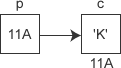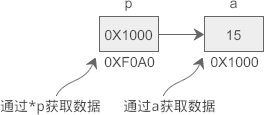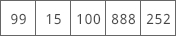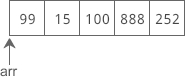# C语言指针学习总结

2019/04/10 10:10

[TOC] 这里对 C 语言的指针进行比较详细的整理总结，参考网络上部分资料整理如下。

# 指针概念

``````int a = 100;
char str = "tanweime.com";
printf("%#X, %p\n", &a, str);

---

0XE42523AC, 0XE4252390
``````

`%#X``%p` 表示以十六进制形式输出，并附带前缀`0X`。a 是一个变量，用来存放整数，需要在前面加`&`来获得它的地址；str 本身就表示字符串的首地址，不需要加`&`

C语言中有一个控制符`%p`，专门用来以十六进制形式输出地址，不过 %p 的输出格式并不统一，有的编译器带`0x`前缀，有的不带

## 一切都是地址

C语言用变量来存储数据，用函数来定义一段可以重复使用的代码，它们最终都要放到内存中才能供 CPU 使用。

CPU 只能通过地址来取得内存中的代码和数据，程序在执行过程中会告知 CPU 要执行的代码以及要读写的数据的地址。如果程序不小心出错，或者开发者有意为之，在 CPU 要写入数据时给它一个代码区域的地址，就会发生内存访问错误。这种内存访问错误会被硬件和操作系统拦截，强制程序崩溃，程序员没有挽救的机会。

CPU 访问内存时需要的是地址，而不是变量名和函数名！变量名和函数名只是地址的一种助记符，当源文件被编译和链接成可执行程序后，它们都会被替换成地址。编译和链接过程的一项重要任务就是找到这些名称所对应的地址。

# 指针变量## 定义指针变量

datatype *name;

datatype *name = value;

`*`表示这是一个指针变量，`datatype`表示该指针变量所指向的数据的类型 。例如：

``````int *p1;
``````

p1 是一个指向 int 类型数据的指针变量，至于 p1 究竟指向哪一份数据，应该由赋予它的值决定。再如：

``````int a = 100;int *p_a = &a;
``````

``````//定义普通变量
float a = 99.5, b = 10.6;char c = '@', d = '#';
//定义指针变量
float *p1 = &a;char *p2 = &c;
//修改指针变量的值
p1 = &b;p2 = &d;
``````

`*`是一个特殊符号，表明一个变量是指针变量，定义 p1、p2 时必须带`*`。而给 p1、p2 赋值时，因为已经知道了它是一个指针变量，就没必要多此一举再带上`*`，后边可以像使用普通变量一样来使用指针变量。也就是说，定义指针变量时必须带`*`，给指针变量赋值时不能带`*`

``````int *a, *b, *c;  //a、b、c 的类型都是 int*
``````

``````int *a, b, c;
``````

## 通过指针变量取得数据

`*pointer`;

``````#include <stdio.h>
int main(){
int a = 15;
int *p = &a;
printf("%d, %d\n", a, *p);  //两种方式都可以输出a的值
return 0;}
````````````int a = 15, b = 99, c = 222;
int *p = &a; //定义指针变量
*p = b;      //通过指针变量修改内存上的数据
c = *p;      //通过指针变量获取内存上的数据
printf("%d, %d, %d, %d\n", a, b, c, *p);
``````

*p 代表的是 a 中的数据，它等价于 a，可以将另外的一份数据赋值给它，也可以将它赋值给另外的一个变量。

`*`在不同的场景下有不同的作用：`*`可以用在指针变量的定义中，表明这是一个指针变量，以和普通变量区分开；使用指针变量时在前面加`*`表示获取指针指向的数据，或者说表示的是指针指向的数据本身。

``````int *p = &a;*p = 100;
``````

``````int *p;p = &a;*p = 100;
``````

``````int x, y, *px = &x, *py = &y;
y = *px + 5;  //表示把x的内容加5并赋给y，*px+5相当于(*px)+5
y = ++*px;  //px的内容加上1之后赋给y，++*px相当于++(*px)
y = *px++;  //相当于y=(*px)++
py = px;  //把一个指针的值赋给另一个指针
``````

## 关于 * 和 & 的谜题

`*&a`可以理解为`*(&a)``&a`表示取变量 a 的地址（等价于 pa），`*(&a)`表示取这个地址上的数据（等价于 *pa），绕来绕去，又回到了原点，`*&a`仍然等价于 a。

`&*pa`可以理解为`&(*pa)``*pa`表示取得 pa 指向的数据（等价于 a），`&(*pa)`表示数据的地址（等价于 &a），所以`&*pa`等价于 pa。

## 对星号`*`的总结

• 表示乘法，例如`int a = 3, b = 5, c; c = a * b;`，这是最容易理解的。
• 表示定义一个指针变量，以和普通变量区分开，例如`int a = 100; int *p = &a;`
• 表示获取指针指向的数据，是一种间接操作，例如`int a, b, *p = &a; *p = 100; b = *p;`

# 指针变量的运算

``````#include <stdio.h>
int main(){
int    a = 10,   *pa = &a, *paa = &a;
double b = 99.9, *pb = &b;
char   c = '@',  *pc = &c;
//最初的值
printf("&a=%#X, &b=%#X, &c=%#X\n", &a, &b, &c);
printf("pa=%#X, pb=%#X, pc=%#X\n", pa, pb, pc);
//加法运算
pa++; pb++; pc++;
printf("pa=%#X, pb=%#X, pc=%#X\n", pa, pb, pc);
//减法运算
pa -= 2; pb -= 2; pc -= 2;
printf("pa=%#X, pb=%#X, pc=%#X\n", pa, pb, pc);
//比较运算
if(pa == paa){
printf("%d\n", *paa);
}else{
printf("%d\n", *pa);
}
return 0;
}
--------

&a=0X28FF44, &b=0X28FF30, &c=0X28FF2B
pa=0X28FF44, pb=0X28FF30, pc=0X28FF2B
pa=0X28FF48, pb=0X28FF38, pc=0X28FF2C
pa=0X28FF40, pb=0X28FF28, pc=0X28FF2A
2686784
``````

# 数组指针``````#include <stdio.h>
int main(){
int arr[] = { 99, 15, 100, 888, 252 };
int len = sizeof(arr) / sizeof(int);  //求数组长度
int i;
for(i=0; i<len; i++){
printf("%d  ", *(arr+i) );  //*(arr+i)等价于arr[i]
}
printf("\n");
return 0;
}
----

99  15  100  888  252
``````

arr 是`int*`类型的指针，每次加 1 时它自身的值会增加 sizeof(int)，加 i 时自身的值会增加 sizeof(int) * i

``````int arr[] = { 99, 15, 100, 888, 252 };int *p = arr;
``````

arr 本身就是一个指针，可以直接赋值给指针变量 p。arr 是数组第 0 个元素的地址，所以`int *p = arr;`也可以写作`int *p = &arr;`。也就是说，arr、p、&arr 这三种写法都是等价的，它们都指向数组第 0 个元素，或者说指向数组的开头。

``````#include <stdio.h>
int main(){
int arr[] = { 99, 15, 100, 888, 252 };
int i, *p = arr, len = sizeof(arr) / sizeof(int);
for(i=0; i<len; i++){
printf("%d  ", *(p+i) );
}
printf("\n");
return 0;
}
``````

## 关于数组指针的谜题

*p++ 等价于 *(p++)，表示先取得第 n 个元素的值，再将 p 指向下一个元素，上面已经进行了详细讲解。

*++p 等价于 *(++p)，会先进行 ++p 运算，使得 p 的值增加，指向下一个元素，整体上相当于 *(p+1)，所以会获得第 n+1 个数组元素的值。

(*p)++ 就非常简单了，会先取得第 n 个元素的值，再对该元素的值加 1。假设 p 指向第 0 个元素，并且第 0 个元素的值为 99，执行完该语句后，第 0 个元素的值就会变为 100。

# 字符串指针

C语言中没有特定的字符串类型，我们通常是将字符串放在一个字符数组中：

``````#include <stdio.h>
#include <string.h>
int main(){
char str[] = "tanweime";
int len = strlen(str), i;
//直接输出字符串
printf("%s\n", str);
//每次输出一个字符
for(i=0; i<len; i++){
printf("%c", str[i]);
}
printf("\n");
return 0;
}
``````

``````char *str = "tanweime";
``````

``````char *str;str = "tanweime";
``````

``````#include <stdio.h>
#include <string.h>
int main(){
char *str = "tanweime";
int len = strlen(str), i;

//直接输出字符串
printf("%s\n", str);
//使用*(str+i)
for(i=0; i<len; i++){
printf("%c", *(str+i));
}
printf("\n");
//使用str[i]
for(i=0; i<len; i++){
printf("%c", str[i]);
}
printf("\n");
return 0;
}
``````

# 指针作为函数参数

``````#include <stdio.h>
void swap(int *p1, int *p2){
int temp;  //临时变量
temp = *p1;
*p1 = *p2;
*p2 = temp;
}
int main(){
int a = 66, b = 99;
swap(&a, &b);
printf("a = %d, b = %d\n", a, b);
return 0;
}
``````

## 用数组作函数参数

``````#include <stdio.h>
int max(int *intArr, int len){
int i, maxValue = intArr;  //假设第0个元素是最大值
for(i=1; i<len; i++){
if(maxValue < intArr[i]){
maxValue = intArr[i];
}
}

return maxValue;
}
int main(){
int nums, i;
int len = sizeof(nums)/sizeof(int);
//读取用户输入的数据并赋值给数组元素
for(i=0; i<len; i++){
scanf("%d", nums+i);
}
printf("Max value is %d!\n", max(nums, len));
return 0;
}
--------
运行结果：
12 55 30 8 93 27↙
Max value is 93!
``````

``````int max(int intArr[], int len){
int i, maxValue = intArr;  //假设第0个元素是最大值
for(i=1; i<len; i++){
if(maxValue < intArr[i]){
maxValue = intArr[i];
}
}
return maxValue;
}
``````

`int intArr[]`虽然定义了一个数组，但没有指定数组长度，好像可以接受任意长度的数组。

`int intArr`这种形式只能说明函数期望用户传递的数组有 6 个元素，并不意味着数组只能有 6 个元素，真正传递的数组可以有少于或多于 6 个的元素。

C语言为什么不允许直接传递数组的所有元素，而必须传递数组指针呢？

# 指针作为返回值

C语言允许函数的返回值是一个指针（地址），我们将这样的函数称为指针函数。下面的例子定义了一个函数 strlong()，用来返回两个字符串中较长的一个

``````#include <stdio.h>
#include <string.h>
char *strlong(char *str1, char *str2){
if(strlen(str1) >= strlen(str2)){
return str1;
}else{
return str2;
}
}
int main(){
char str1, str2, *str;
gets(str1);
gets(str2);
str = strlong(str1, str2);
printf("Longer string: %s\n", str);
return 0;
}
``````

``````#include <stdio.h>
int *func(){
int n = 100;
return &n;
}
int main(){
int *p = func(), n;
n = *p;
printf("value = %d\n", n);
return 0;
}
``````

# 二级指针

``````int a =100;
int *p1 = &a;
int **p2 = &p1;
``````

``````int ***p3 = &p2;
``````

``````int ****p4 = &p3;
``````

``````#include <stdio.h>
int main(){
int a =100;
int *p1 = &a;
int **p2 = &p1;
int ***p3 = &p2;
printf("%d, %d, %d, %d\n", a, *p1, **p2, ***p3);
printf("&p2 = %#X, p3 = %#X\n", &p2, p3);
printf("&p1 = %#X, p2 = %#X, *p3 = %#X\n", &p1, p2, *p3);
printf(" &a = %#X, p1 = %#X, *p2 = %#X, **p3 = %#X\n", &a, p1, *p2, **p3);
return 0;
}
------
100, 100, 100, 100
&p2 = 0XE19322F8, p3 = 0XE19322F8
&p1 = 0XE1932300, p2 = 0XE1932300, *p3 = 0XE1932300
&twa = 0XE193230C, p1 = 0XE193230C, *p2 = 0XE193230C, **p3 = 0XE193230C
``````

# 指针数组

dataType *arrayName[length];

`[ ]`的优先级高于`*`，该定义形式应该理解为：

dataType *(arrayName[length]);

``````#include <stdio.h>
int main(){
int a = 16, b = 932, c = 100;
//定义一个指针数组
int *arr = {&a, &b, &c};//也可以不指定长度，直接写作 int *parr[]
//定义一个指向指针数组的指针
int **parr = arr;
printf("%d, %d, %d\n", *arr, *arr, *arr);
printf("%d, %d, %d\n", **(parr+0), **(parr+1), **(parr+2));
return 0;
}
------
运行结果：
16, 932, 100
16, 932, 100
``````

``````#include <stdio.h>
int main(){
char *str = {
"tanwei",
"谭巍",
"C Language"
};
printf("%s\n%s\n%s\n", str, str, str);
return 0;
}
``````

``````#include <stdio.h>
int main(){
char *str0 = "tanwei";
char *str1 = "谭巍";
char *str2 = "C Language";
char *str = {str0, str1, str2};
printf("%s\n%s\n%s\n", str, str, str);
return 0;
}
``````

# 指针与二维数组

int a = { {0, 1, 2, 3}, {4, 5, 6, 7}, {8, 9, 10, 11} };

``````0   1   2   3
4   5   6   7
8   9  10  11
``````

C语言允许把一个二维数组分解成多个一维数组来处理。对于数组 a，它可以分解成三个一维数组，即 a、a、a。每一个一维数组又包含了 4 个元素，例如 a 包含 a、a、a、a。

int (*p) = a;

`[ ]`的优先级高于`*``( )`是必须要加的，如果赤裸裸地写作`int *p`，那么应该理解为`int *(p)`，p 就成了一个指针数组，而不是二维数组指针。

1. `p`指向数组 a 的开头，也即第 0 行；`p+1`前进一行，指向第 1 行。

2. `*(p+1)`表示取地址上的数据，也就是整个第 1 行数据。注意是一行数据，是多个数据，不是第 1 行中的第 0 个元素，下面的运行结果有力地证明了这一点：

``````#include <stdio.h>
int main(){
int a = { {0, 1, 2, 3}, {4, 5, 6, 7}, {8, 9, 10, 11} };
int (*p) = a;
printf("%d\n", sizeof(*(p+1)));
return 0;
}
---
16
``````
1. `*(p+1)+1`表示第 1 行第 1 个元素的地址。如何理解呢？

`*(p+1)`单独使用时表示的是第 1 行数据，放在表达式中会被转换为第 1 行数据的首地址，也就是第 1 行第 0 个元素的地址，因为使用整行数据没有实际的含义，编译器遇到这种情况都会转换为指向该行第 0 个元素的指针；就像一维数组的名字，在定义时或者和 sizeof、& 一起使用时才表示整个数组，出现在表达式中就会被转换为指向数组第 0 个元素的指针。

1. `*(*(p+1)+1)`表示第 1 行第 1 个元素的值。很明显，增加一个 * 表示取地址上的数据。

``````a+i == p+i
a[i] == p[i] == *(a+i) == *(p+i)
a[i][j] == p[i][j] == *(a[i]+j) == *(p[i]+j) == *(*(a+i)+j) == *(*(p+i)+j)
``````
``````#include <stdio.h>
int main(){
int a={0,1,2,3,4,5,6,7,8,9,10,11};
int(*p);
int i,j;
p=a;
for(i=0; i<3; i++){
for(j=0; j<4; j++) printf("%2d  ",*(*(p+i)+j));
printf("\n");
}
return 0;
}
``````

## 指针数组和二维数组指针的区别

``````int *(p1);  //指针数组，可以去掉括号直接写作 int *p1;int (*p2);  //二维数组指针，不能去掉括号
``````

# 函数指针

returnType (*pointerName)(param list);

returnType 为函数返回值类型，pointerNmae 为指针名称，param list 为函数参数列表。参数列表中可以同时给出参数的类型和名称，也可以只给出参数的类型，省略参数的名称，这一点和函数原型非常类似。

``````#include <stdio.h>
//返回两个数中较大的一个
int max(int a, int b){
return a>b ? a : b;
}
int main(){
int x, y, maxval;
//定义函数指针
int (*pmax)(int, int) = max;  //也可以写作int (*pmax)(int a, int b)
printf("Input two numbers:");
scanf("%d %d", &x, &y);
maxval = (*pmax)(x, y);
printf("Max value: %d\n", maxval);
return 0;
}
``````

# 总结

int *p; p 可以指向 int 类型的数据，也可以指向类似 int arr[n] 的数组。
int **p; p 为二级指针，指向 int * 类型的数据。
int *p[n]; p 为指针数组。[ ] 的优先级高于 *，所以应该理解为 int *(p[n]);
int (*p)[n]; p 为二维数组指针。
int *p(); p 是一个函数，它的返回值类型为 int *。
int (*p)(); p 是一个函数指针，指向原型为 int func() 的函数。
1. 指针变量可以进行加减运算，例如`p++``p+i``p-=i`。指针变量的加减运算并不是简单的加上或减去一个整数，而是跟指针指向的数据类型有关。

2. 给指针变量赋值时，要将一份数据的地址赋给它，不能直接赋给一个整数，例如`int *p = 1000;`是没有意义的，使用过程中一般会导致程序崩溃。

3. 使用指针变量之前一定要初始化，否则就不能确定指针指向哪里，如果它指向的内存没有使用权限，程序就崩溃了。对于暂时没有指向的指针，建议赋值`NULL`

4. 两个指针变量可以相减。如果两个指针变量指向同一个数组中的某个元素，那么相减的结果就是两个指针之间相差的元素个数。

5. 数组也是有类型的，数组名的本意是表示一组类型相同的数据。在定义数组时，或者和 sizeof、& 运算符一起使用时数组名才表示整个数组，表达式中的数组名会被转换为一个指向数组的指针。

0
0 收藏

### 作者的其它热门文章0 评论
0 收藏
0